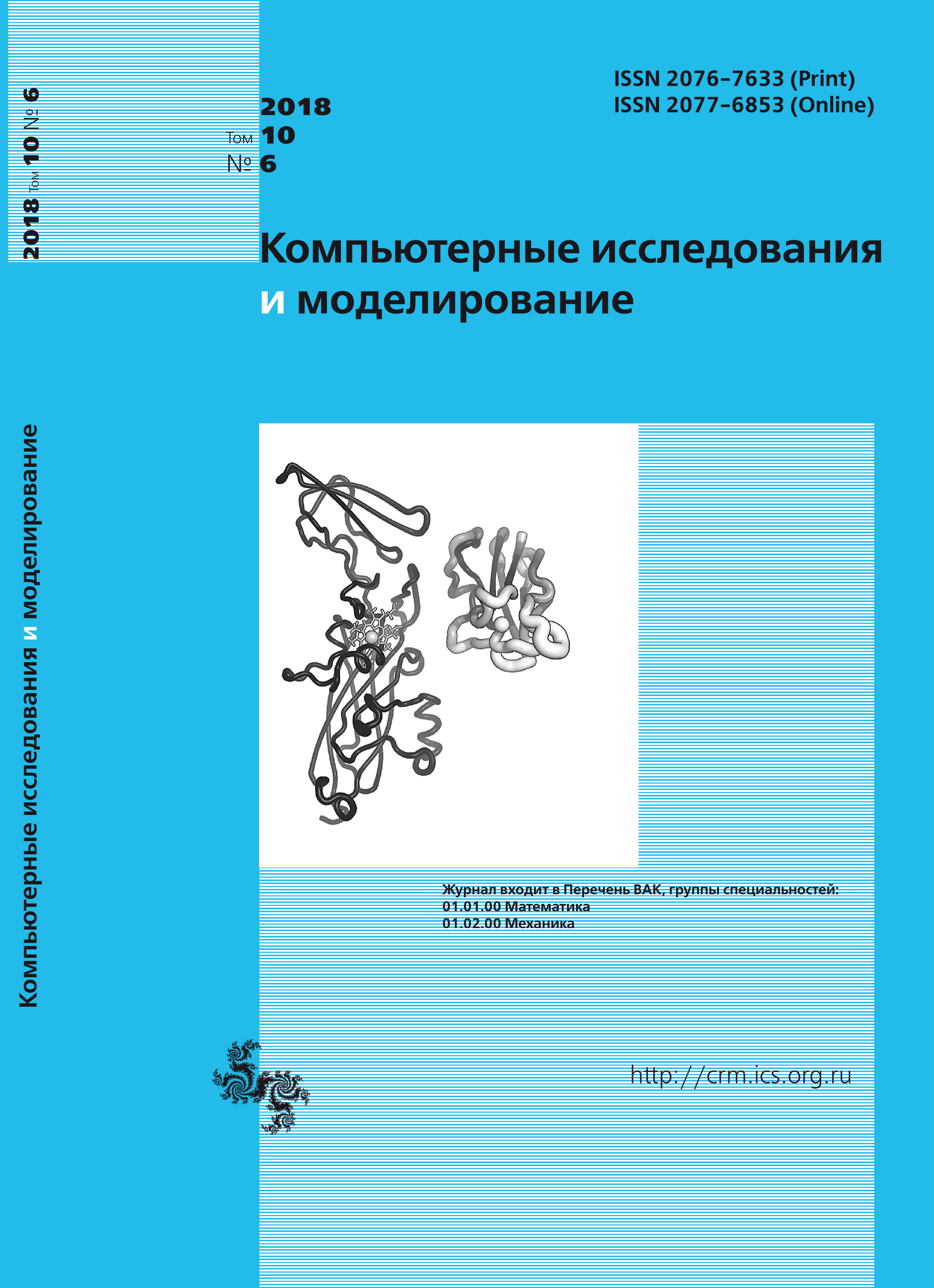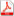Issue 6, 2018 Vol. 10

# All issues

The global rate of convergence for optimal tensor methods in smooth convex optimizationpdf (180K)  / List of references

In this work we consider Monteiro – Svaiter accelerated hybrid proximal extragradient (A-HPE) framework and accelerated Newton proximal extragradient (A-NPE) framework. The last framework contains an optimal method for rather smooth convex optimization problems with second-order oracle. We generalize A-NPE framework for higher order derivative oracle (schemes). We replace Newton’s type step in A-NPE that was used for auxiliary problem by Newton’s regularized (tensor) type step (Yu. Nesterov, 2018). Moreover we generalize large step A-HPE/A-NPE framework by replacing Monteiro – Svaiter’s large step condition so that this framework could work for high-order schemes. The main contribution of the paper is as follows: we propose optimal highorder methods for convex optimization problems. As far as we know for that moment there exist only zero, first and second order optimal methods that work according to the lower bounds. For higher order schemes there exists a gap between the lower bounds (Arjevani, Shamir, Shiff, 2017) and existing high-order (tensor) methods (Nesterov – Polyak, 2006; Yu.Nesterov, 2008; M. Baes, 2009; Yu.Nesterov, 2018). Asymptotically the ratio of the rates of convergences for the best existing methods and lower bounds is about 1.5. In this work we eliminate this gap and show that lower bounds are tight. We also consider rather smooth strongly convex optimization problems and show how to generalize the proposed methods to this case. The basic idea is to use restart technique until iteration sequence reach the region of quadratic convergence of Newton method and then use Newton method. One can show that the considered method converges with optimal rates up to a logarithmic factor. Note, that proposed in this work technique can be generalized in the case when we can’t solve auxiliary problem exactly, moreover we can’t even calculate the derivatives of the functional exactly. Moreover, the proposed technique can be generalized to the composite optimization problems and in particular to the constraint convex optimization problems. We also formulate a list of open questions that arise around the main result of this paper (optimal universal method of high order e.t.c.).

Keywords: Newton method, Hesse matrix, lower bounds, tensor methods, proximal fast gradient descent
Citation in English: Gasnikov A.V., Gorbunov E.A., Kovalev D.A., Mohammed A.A., Chernousova E.O. The global rate of convergence for optimal tensor methods in smooth convex optimization // Computer Research and Modeling, 2018, vol. 10, no. 6, pp. 737-753
Citation in English: Gasnikov A.V., Gorbunov E.A., Kovalev D.A., Mohammed A.A., Chernousova E.O. The global rate of convergence for optimal tensor methods in smooth convex optimization // Computer Research and Modeling, 2018, vol. 10, no. 6, pp. 737-753
DOI: 10.20537/2076-7633-2018-10-6-737-753
Views (last year): 75.

Full-text version of the journal is also available on the web site of the scientific electronic library eLIBRARY.RU

The journal is included in the Russian Science Citation Index

The journal is included in the List of Russian peer-reviewed journals publishing the main research results of PhD and doctoral dissertations.

International Interdisciplinary Conference "Mathematics. Computing. Education"

The journal is included in the RSCI

Indexed in Scopus